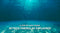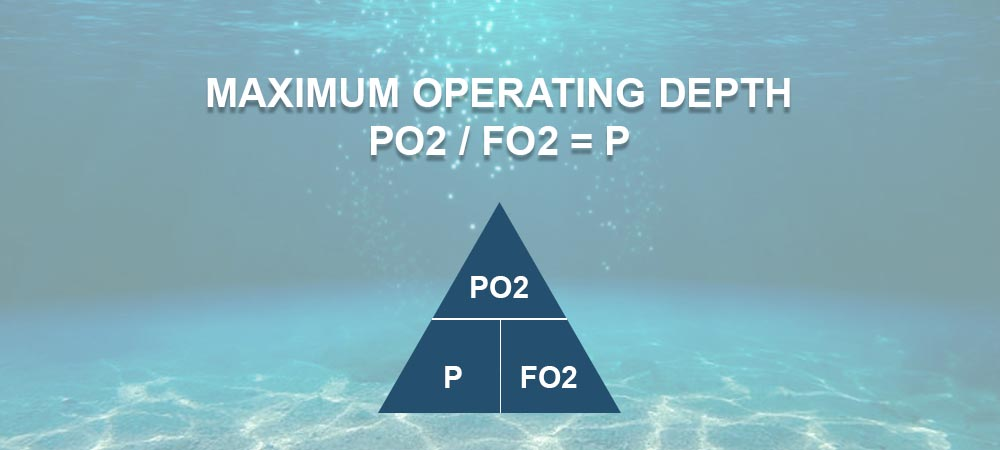## A step by step guide to Nitrox formulas

As recreational divers we are told to always keep the partial pressure (PO2) to below 1.4 ata when you dive. If you are already a nitrox diver, then some of this should be familiar to you, if you certified nitrox diver, then this may not all make sense yet.

During your training your instructor may even have been introduced to “The Pressure T”. This is not required, but it is a useful way explain what is happening on your dive computer. It is also useful to know how the Pressure T works to work out some really useful information before your dive, even without having a dive computer to hand.

#### Let's get started

These should answer most answers you have, but we understand that there are always specific questions about unique circumstances, our team can help, so reach out to them.### Using the Pressure Triangle

The Pressure T, Pressure Triangle or Dalton's Diamond can be used to help you answer a number of questions you may have as a nitrox diver. The three things that the pressure triangle can give you is:

• The best mix for given depth dive
• The partial pressure of the gas
• The maximum depth a gas can safely be used

Although it may look a little complicated at first, it is really pretty straight forward. You simply cover up the item you are looking for the answer to and you can find the formula you need. However, you need to understand what the letters mean:

• PO2 = Partial Pressure of Oxygen
• P = Pressure in Bar
• FO2 = Fraction of oxygen in the blend

Then you can see that there is three formulas from above:

• PO2 = P x FO2
• FO2 = PO2 / P
• P =PO2 / F02

If you are following this, then great, if not, don't worry, we are about to explain each in detail.### Maximum Operating Depth Formula

This allows you to choose establish the maximum depth a gas can be safely breathed during a dive. This is worked out as follows:

• Partial Pressure of Oxygen / Fraction of Oxygen = Maximum Depth
For a dive where we want to not exceed our 1.4 maximum pressure of oxygen with a 32% nitrox blend this would be your formula:
• 1.4 / 0.32 = 4.375

This is the absolute pressure, therefore, this means we can dive to 33.75m with a 32% nitrox blend.

A good example of this is on a liveaboard when they top up your gas after the first dive on air and you analyse it at 29%.

This calculation will let you work out your Maximum Operating Depth (MOD) 1.4 ata and your Contingency Operating Depth (COD) substituting the 1.4 for 1.6 into the PO2 in the formula.

### Partial Pressure of Gas Formula

To find out the Partial Pressure of Oxygen (PO2) for a given depth and blend of gas you would work this out as follows:

• Fraction of Oxygen x Pressure in Bar = Pressure of Oxygen

For the same 25m wreck dive but choosing to use a 36% nitrox blend this would work out as follows:

• 0.36 / 3.5 = 1.26

This means that partial pressure for a 36% nitrox blend being breathed at 25m would be within our maximum allowable at 1.26 PO2.### Best Mix Formula

This is one of the most commonly used formulas. This allows you to choose the best nitrox mix for a given depth of dive to maximise your bottom time during your dive. This is worked out as follows:

• Partial Pressure of Oxygen / Pressure in Bar = Best Blend Of Nitrox

For a 25m wreck dive this would be 3.5 bar of pressure and the Partial Pressure of Oxygen we use as recreational divers is 1.4 for our maximum pressure of oxygen.

• 1.4 / 3.5 = 0.4

This means that diving a 40% nitrox blend at 25m would be our best choice of nitrox blend.

We’d use this if we are planning to dive to a specific depth to see something in particular. We’d want the best (highest) FO2 to let us stay longer but compromise that to give us a safe PO2